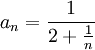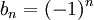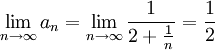# Convergence of Infinite Sequences Example

Previous: Convergence of Infinite Sequences

Next: Relationship to Limits of Functions

## Example

Determine whether the sequencesandconverge.

## Complete Solution

### The Sequence an

Using the definition of convergence of an infinite sequence, we would evaluate the following limit:Because this limit evaluates to a single finite number, the sequence converges.

### The Sequence bn

While the sequence an converges to 1/2, bn does not converge because its terms do not approach any number. Instead, the terms in the sequence oscillate between -1 and +1.

The sequence bn does not converge to a unique number, and so bn does not converge.

Previous: Convergence of Infinite Sequences

Next: Relationship to Limits of Functions